• Call Now

1800-102-2727•

# Reverberation

When you attend an assembly inside the school auditorium, you definitely observe how the voice of the orator appears changed from the original.And the sound produced by the Instruments in a closed room appears very different when compared to its original sound. Ever wondered why? This phenomenon is called reverberation of sound.

Table of content

## What is reverberation?

Reverberation is the persistence of sound after it has been stopped as a result of multiple reflections from surfaces such as room walls, furniture, air, etc. within a closed surface. These reflections are created with each reflection and vanishes gradually as they are absorbed by the surfaces of objects in the space enclosed.

Reverberation is the persistence of sound after it has been stopped due to multiple reflections from surfaces such as room walls, furniture, air, etc. within a closed surface. These reflections are created with each reflection and vanishes gradually as they are absorbed by the surfaces of objects in the space enclosed.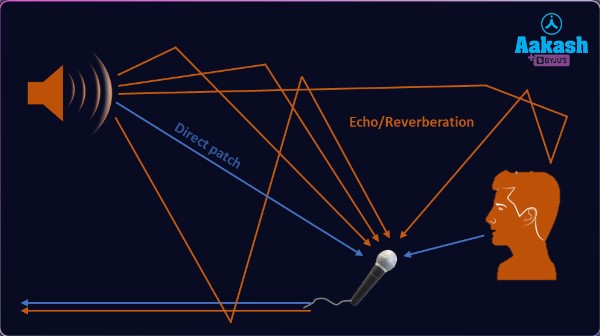Reverberation is the same as the echo, but the distance between the source and obstacle through which it gets reflected is less in case of this reverberation. The Numerical calculation of the reverberation is mainly done with the help of the parameter called reverberation time. Reverberation time is defined as time duration when the sound decays by about 60 decibels starting from the initial level. In the process of reverberation, the time delay be less than 0.1 second i.e. the reflected wave reaches the observer in less than 0.1 seconds.That delay in the perception of the sound and the original sound is very less and whereas the original sound will be still in the mind when this reflected sound is heard.

Reverberation is helpful when it comes to musical symphonies and orchestra halls, when a good amount of reverberation is present, the sound quality gets enhanced drastically. Because of this sound engineers are appointed during the construction of these halls.

When a room has nearly no sound-absorbing Body or surface like the wall, roof, and the floor, the sound bounces back between the surfaces, and also it takes a long time as the sound dies. In such a room, the listener will have problems . That's why he tends to hear both the direct sound and the repeated reflected sound waves.If reverberations are more excessive, the sound is said to run together with a mere loss of articulation, and it becomes muddy and also garbled.

## How do we Measure Reverberation?

one can measure the reverberation time by using a sound level meter and loudspeaker. Furthermore, one can also calculate the reverberation time by using the formula of Sabins. Moreover, the basis of this equation is the volume of the space and the total amount of absorption within a space.

To calculate the sabin values, we use the volume (length x width x height) of the room as well as the surface materials.One can take help from a table that contains the sabins at each octave band frequency for materials used in construction. Finally, the addition of the sound absorption to the calculation can take place.

Reverberation time can be measured with the help of a sound level meter and loudspeaker.

Also reverberation time can be calculated by the Sabin formula. According to the Sabin formula, reservation time depends upon the volume of the space and sound absorption in that space.

For the measure of reverberation, first we calculate sabin value for all surfaces, after summation of sabins values total absorption is found and then by using the sabine formula Reverberation can be calculated.

## Formula of Reverberation

Formula for Sabins:

a = Σ S α

Where:

S = surface area ( in feet squared)

α = sound absorption coefficient

After the calculation of Sabins, Now use the Sabine Formula for calculating the reverberation time.

Sabine Formula: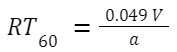Where:

RT60 = Reverberation Time (in second)

V = volume of the space (in feet cubed)

a = sabins (total room absorption that is present at a given frequency)

## Practice problem

Q 1. Find reverberation time for a room of dimension 40 x 30 x 20 ft3 having average absorption coefficient of 0.15.

Answer: Given L=40, W=30, H=20, a=0.15

Volume of the hall, V=40 x 30 x 20=24000 ft3

Surface area of Room, S=2(40 x 30+30 x 20+20 x 40)

S=2 x (1200+600+800)=5200 ft2

Total average absorption, aS=0.15 x 5200 ft2=780 ft2

Reverberation time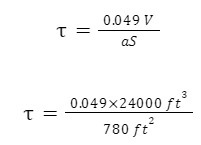=1.51 s

Q 2,. In the above problem, How much area we should put an absorbing material of absorption coefficient, 0.20, to reduce its reverberation time to 1.2 s?

Answer: Given a= 0.20 , =1.2 s

Total average absorption , aS=0.15 x 5200 -A+0.20 A=780 +0.05 A

Reverberation time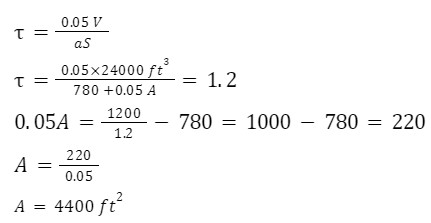Q 3. In a College lecture hall (30 ft x 20 ft x 15 ft) is heavily damped with an absorption coefficient 0.3. Calculate its reverberation time.

Answer: Given L=30 ft , W=20 ft, H= 15 ft, a=0.3

Reverberation time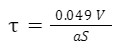Volume of the hall, V=30 x 20 x 15=9000 ft3

Surface area of Room, S=2(30 x 20+20 x 15+15 x 30)=1350 ft2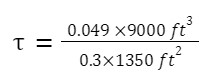=1.09 s

Q 4. In a hall of 70 x 40 x 15 ft3 has surface of absorption coefficient, 0.1, and a capacity of an audience of 100 adults (each having an absorption of 4.7 ft2OWU), find reverberation time of the hall.

Answer: Given L=70, W=40, H=15, a=0.1, n=100

a1 S1=0.12(70 x 40+40 x 15+15 x 70)+4.7100

a1 S1=0.1 x 8900+4.7 x 100=890+470=1360 ft2 OWU

Reverberation time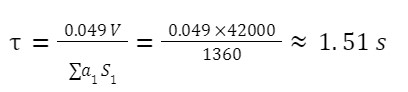## FAQs

Q 1. What is reverberation?
Reverberation refers to the combination of multiple echoes that would occur in a big room or hall. it exists in all indoor spaces but not in most outdoor places.

Q 2. What is reverberation time?
Time for which the acoustic energy will be present in the room is referred to as reverberation time.Or the time it takes for the energy to diminish by a factor of one million (60 dB).

Q 3. Write one difference between echo and reverberation?
An echo takes longer to return back to our hearing than reverberation.

Q 4. Write the name of reverberation devices?

2. Plate reverberator

 Type of Waves,Terms related to Waves,Practice Problems, FAQs Transverse Wave-definition,Practice problem,FAQs Longitudinal waves-Practice Problems,FAQs Progressive wave : Displacement RelationTalk to our expert
Resend OTP Timer =
By submitting up, I agree to receive all the Whatsapp communication on my registered number and Aakash terms and conditions and privacy policy